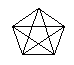Email us to get an instant 20% discount on highly effective K-12 Math & English kwizNET Programs!

#### Online Quiz (WorksheetABCD)

Questions Per Quiz = 2 4 6 8 10

### MEAP Preparation - Grade 6 Mathematics4.22 Diagonals

 A diagonal is a line segment that is not a side and that joins two vertices of a polygon. Directions: Answer the following. Also draw a triangle, square and rectangle and show its diagonals.
 Q 1: A hexagon has ______diagonals.896 Q 2: From the figure can you find out the number of diagonals for a 10 sided polygon?372735 Q 3: The figure gives the number of sides and their diagonals. What is the pattern?2,4,5,63,4,5,6,71,2,3,4 Q 4: A pentagon has ______diagonals.6574 Q 5: The diagonals of a rhombus areperpendicular to each otherparallel to each other Q 6: A quadrilateral has ___ diagonals.432 Question 7: This question is available to subscribers only! Question 8: This question is available to subscribers only!